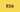# Look for ES6 important concepts in 2019

To learn how to use ES6, it is of very much importance that you should know what are the latest features or concepts are and that would help you to use ES6 in any kind of javascript projects.

### What is ES6?

ES6 also known as ECMAScript 6 and developers usually call it Javascript 6.

### Let us see some of the new features in ES6:

• let
• const
• Arrow Functions
• Array find function
• Array findIndex function

### Let's dive into each one of the above:

• Let

• With let you can declare a variable within a block scope.
``````		{
var testing = 'I have the complete scope;

{
let testing = 'My scope is limited';
}

// testing value here is `I have the complete scope`
}``````
• The value of variable can be changed further in the code within the scope.
• Const

• In ES6 the const keyword can be used to create a variable with constant value, which ofcourse cannot be changed.
• They posses the same properties of `let` except the value of it cannout be modified later in the code.
• Arrow Functions

• A much shorter syntax for writing functions in javascript.

``````// In ES5
var arrowTest = function(a,b){
return a-b;
}
// In ES6
const arrowSet = (a,b)=> a-b;``````
• Use of `const` keyword is more preferred when creating a arrow function and assigning it over the `var` keyword, because a function expression is always constant value.
• Arrow functions do not have `this`.
• The `return` keyword can be avoided when returing in a single line of statement but can be used when put inside curly brakets.

``````const x = (a,b) => a * b;   // can directly return

const y = (x,y) => {return x * y};  // need curly brakets to return 		``````
• Find function in ES6

• Accepts a function in the parameter which contains the condition or logic to find the value in the array and returns the first element to match the parameter returned condition.

``````const arr = [1,4,40,60];
console.log(arr.find(x => x > 50));  // 60``````
• The parameter function takes 3 arguments
• Item value
• Item Index
• Array itself
• Find Index function

• Accepts a function in the parameter which returns the index of the first matched logic/condition in parameter function.
``````		const arr = [1,6,78,45,67];
console.log(arr.findIndex(x => x > 45)); // 2``````